T H E   W H I T E   H O U S E Appendix A Help Site Map Text Only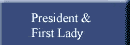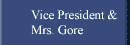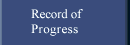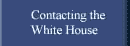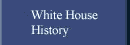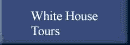# Appendix A

## Summary of Cancer Risk Derivations for MTBE - A Screening Evaluation

Jennifer Jinot: Quantitative Risk Methods Group
National Center for Environmental Assessment
U.S. EPA, Washington, DC
29 November, 1995

The following information has not been EPA peer reviewed and so should not be construed as a final EPA position. This summary is being provided for use in the Interagency Short-Term Health Effects Assessment of Oxygenated Fuels. Estimates are presented using two significant figures to minimize compounding round-off errors during further calculations

Note:

• 1 ppm MTBE = 1.05 mg/kg/d @ 20°C = 3.67 mg/m3
MTBE MW = 88.15

### SUMMARY OF RISK ESTIMATES FOR METHYL TERTIARY BUTYL ETHER (MTBE) BASED ON MOUSE LIVER TUMORS

inhalation upper bound unit risk: 1.5E-7 per ug/m3 (5.3E-4 per mg/kg/d, 5.5E-4 per ppm)

extrapolation method: linearized multistage (GLOBAL86), extra risk

ED10: 480 mg/kg/d, 460 ppm

### DOSE-RESPONSE DATA

tumor type: hepatocellular adenoma and/or carcinoma
test animal: CD-1 mouse
route: inhalation
reference: Burleigh-Flayer et al. (1992)

#### female

exposure
human equivalent
exposure (ppm)
tumor incidence
0 0 2/50 0/50 2/50
400 70 1/50 1/50 2/50
3000 540 2/50 0/50 2/50
8000 1430 10/50 1/50 11/50

#### male

exposure
human equivalent
exposure (ppm)
tumor incidence
0 0 11/47 2/42 12/47
400 70 11/47 4/45 12/47
3000 540 9/46 3/41 12/46
8000 1430 12/37 8/34 16/37

1. The unit risk is considered to be a plausible upper bound on the increased cancer risk from lifetime inhalation of MTBE. It represents the 95% upper confidence limit (UCL) on the linear term of the multistage model.

2. Due to increased early mortality in the male mice, the denominators in the male mouse tumor incidences were corrected to include only those animals alive at the time of the first observed tumor, week 49 for adenomas and week 63 for carcinomas.

3. The inhalation unit risk estimate of 1.5E-7 per ug/m3 reflects an averaging of the unit risk based on the combined tumor incidence in the females (3.4E-4 per ppm) and the unit risk based on the combined tumor incidence in the males (7.7E-4 per ppm), i.e. 5.5E-4 per ppm, and a conversion of units from ppm to ug/m3 [1 ppm = 1.05 mg/kg/day = 3.67E+3 ug/m3].

4. These tumor incidences are from an 18-month study. The unit risk estimate was adjusted to reflect a full lifetime (24 months). The lifetime adjustment factor is 2.37 (i.e., (24/18)3).

5. Human equivalent daily exposures were calculated by adjusting animal exposures of 6 hours/day, 5 days/week to 24 hours/day, 7 days/week and assuming ppm equivalence between species for exposure (i.e., scaling for respiration and metabolism are assumed to cancel out).

6. If the unit risk were estimated from only the hepatocellular carcinomas in male mice, the estimate would decrease by less than a factor of 2.

7. Anesthetic effects were observed in the mice at the 3000 and 8000 ppm exposure levels of this ether. Such a response would imply a decreased inhalation rate, and consequently a decreased dose. Without the necessary data to correct for this effect, the potency (unit risk) is underestimated by an unknown amount.

8. If one is interested in central tendency estimates rather than upper bounds, one generally considers maximum likelihood estimates (MLEs) of the parameters of interest. The MLE of the unit risk (generally taken to be the MLE of the linear term) from the multistage model, however, can be unstable (i.e., highly sensitive to small changes in the input data). In addition, under certain circumstances, the computer algorithm of the model cannot generate a legitimate risk estimate (specifically, when the algorithm generates a coefficient that is effectively zero for the linear term, because a linear term is required under multistage theory).

The computer algorithm for the linearized multistage model (LMS) was unable to provide an MLE of the unit risk for these mouse liver tumor data.

One can nonetheless generate MLEs of excess cancer risk for specific exposures (these MLEs reflect the MLE estimates of the full parameter set, not just the linear term of the model). Table A1 below compares MLEs and 95% upper confidence limits (UCLs) for various exposures under the given model (in this case, a 3-stage model). It is important to realize, however, that the MLEs are highly sensitive to small changes in the incidence data, while the 95% UCLs are more stable (See the examples in comment #6 of the section on risk estimates based on the male rat kidney tumor data). Given the instability in the MLEs at low exposures, they are not considered reliable for estimation of human risk.

#### Table A1. 95% UCLs and MLEs for excess cancer risk from the mouse liver tumor data, using the LMS model

daily exposure (ppm)
excess liftime cancer risk
95% UCL MLE
1 5.5E-4 (unit risk) 2.0E-10
0.1 5.5E-5 2.0E-13
0.01 5.5E-6 <E-16
0.001 5.5E-7 <E-16
0.0001 5.5E-8 <E-16

9. To compare the potency of a carcinogen with that of other carcinogens, the Agency has sometimes used "benchmark" methods, specifically, the MLE of the dose associated with an increased cancer risk of 10 percent (the cancer ED10 benchmark). This method is used for ranking because comparisons can be based on estimates that are (1) within the range of experimental observation, (2) compatible with different dose-response models, and (3) estimates of central tendency rather than statistical bounds.

For MTBE, the cancer ED10 benchmark from the mouse liver tumor data is 480 mg/kg/d, estimated by averaging ED10 benchmarks from the two data sets used for estimating the cancer unit risk and adjusting for full lifetime. For comparison, cancer ED10 benchmarks for 80 other Clean Air Act hazardous air pollutants range from 0.0000015 mg/kg/d (most potent) to 80 mg/kg/d (least potent).

Benchmark methods can also provide a common approach for cancer and noncancer risk assessment. As noncancer benchmarks for MTBE become available, they can be compared with the cancer ED10 benchmark of 480 mg/kg/d.

### INHALATION RISK ESTIMATE FOR METHYL TERTIARY BUTYL ETHER (MTBE) BASED ON MALE RAT KIDNEY TUMORS

inhalation upper bound unit risk: 1.7E-7 per ug/m3 (5.9E-4 per mg/kg/d, 6.2E-4 per ppm)

extrapolation method: linearized multistage (Global 86), extra risk

ED10: 350 mg/kg/d, 330 ppm

### DOSE-RESPONSE DATA

tumor type: renal tubular cell adenomas and carcinomas
test animal: F344 male rat
route: inhalation
reference: Chun et al. (1992)

exposure (ppm)
human equivalent
exposure (ppm)
tumor incidence
0 0 1/50 1/35
400 70 0/50 0/32
3000 540 8/50 8/31
8000 1430 3/50 3/20

1. The unit risk is considered to be a plausible upper bound on the increased cancer risk from lifetime inhalation of MTBE. It represents the 95% upper confidence limit (UCL) on the linear term of the multistage model.

2. The unit risk and ED10 values presented above are based on survival-adjusted tumor incidence rates. Survival-adjusted rates were used to attempt to control for excess early mortality (attributed to MTBE-induced chronic progressive nephropathy) in the two highest exposure groups in the second year of the bioassay. In addition, the highest exposure group was dropped from the quantitative risk analysis because the early mortality was especially extreme (this exposure group was terminated at 82 weeks). The survival-adjustment method was to weight each tumor-less animal by its fractional survival time (number of days on study divided by 724 days) raised to the power 3, to reflect the fact that animals are at greater risk of cancer at older ages. Animals with tumors were given a weight of 1. The sum of the weights of all of the animals in an exposure group yields the effective survival-adjusted denominator. (See Portier and Bailer (1989) for a discussion of this survival-adjustment technique.)

An attempt was also made to use a time-to-tumor model (linearized multistage Weibull model) to take into account the early mortality; however, risk estimates produced using this model did not provide a good fit to the observed low-dose animal response.

(The Weibull risk estimate was 1.8E-2 per mg/kg/d).

3. Human equivalent daily exposures were calculated by adjusting animal exposures of 6 hours/day, 5 days/week to 24 hours/day, 7 days/week and assuming ppm equivalence between species for exposure.

4. Anesthetic effects were observed in the rats at the 3000 and 8000 ppm exposure levels of this ether. Such a response would imply a decreased inhalation rate, and consequently a decreased dose. Without the necessary data to correct for this effect, the potency (unit risk) is underestimated by an unknown amount.

5. If one is interested in central tendency estimates rather than upper bounds, one generally considers maximum likelihood estimates (MLEs) of the parameters of interest. The MLE of the unit risk (generally taken to be the MLE of the linear term) from the multistage model, however, can be unstable (i.e., highly sensitive to small changes in the input data). In addition, under certain circumstances, the computer algorithm of the model cannot generate a legitimate risk estimate (specifically, when the algorithm generates a coefficient that is effectively zero for the linear term, because a linear term is required under multistage theory). The computer algorithm for the linearized multistage model was unable to provide an MLE of the unit risk for these male rat kidney tumor data.

One can nonetheless generate MLEs of excess cancer risk for specific exposures (these MLEs reflect the MLE estimates of the full parameter set, not just the linear term of the model). Table A2 below compares MLEs and 95% upper confidence limits (UCLs) for various exposures under the given model (in this case, a 2-stage model). It is important to realize, however, that the MLEs are highly sensitive to small changes in the incidence data, while the 95% UCLs are more stable (See the examples in #6 below). Given the instability in the MLEs at low exposures, they are not considered reliable for estimation of human risk.

#### Table A2. 95% UCLs and MLEs for excess cancer risk from the male rat kidney tumor data, using the LMS model

daily exposure (ppm)
excess lifetime cancer risk
95% UCL MLE
1 6.2E-4 (unit risk) 9.6E-7
0.1 6.2E-5 9.6E-9
0.01 6.2E-6 9.6E-11
0.001 6.2E-7 9.6E-13
0.0001 6.2E-8 9.7E-15

6. The following examples illustrate the sensitivity of the MLE of excess risk at low exposures to small variations in the incidence data, which suffer from statistical power limitations because of the small number of animals used.

In addition to the rat kidney adenomas and carcinomas reported in the incidence rates above (1/35, 0/32, 8/31), there were 2 "preneoplastic" lesions observed only in the 400 ppm exposure group. These preneoplastic lesions are considered to be part of a continuum of stages in the progression of kidney cancer. As a hypothetical example, if these lesions had progressed to adenomas and been included in the rat kidney tumor incidence rates (resulting in incidence rates of 1/35, 2/32, 8/31), the linearized multistage model would have generated MLEs much more comparable to the 95% UCLs, as shown in Table A3 below, a difference of several orders of magnitude from the MLE results in Table A2, while the 95% UCLs would have hardly changed from the results in Table A2. (In this hypothetical example, the LMS algorithm generates a two-stage model.)

#### Table A3. 95% UCLs and MLEs for excess cancer risk from hypothetical incidence data, using the LMS model - example #1

daily exposure (ppm)
excessive lifetime cancer risk
95% UCL MLE
1 8.8E-4 5.0E-4
0.1 8.9E-5 5.0E-5
0.01 8.9E-6 5.0E-6
0.001 8.9E-7 5.0E-7
0.0001 8.9E-8 5.0E-8

As a second hypothetical example, if the "background" tumor which occurred in the control group had instead occurred in the 400 ppm exposure group (resulting in incidence rates of 0/35, 1/32, 8/31), the linearized multistage model would have generated MLEs much more comparable to the 95% UCLs, as shown in Table A4 below, a difference of several orders of magnitude from the MLE results in Table A2, while the 95% UCLs would have hardly changed from the results in Table A2. (In this hypothetical example, the LMS algorithm generates a two-stage model.)

#### Table A4. 95% UCLs and MLEs for excess cancer risk from hypothetical incidence data, using the LMS model - example #2

daily exposure (ppm)
excess lifetime cancer risk
95% UCL MLE
1 8.9E-4 4.3E-4
0.1 8.9E-5 4.3E-5
0.01 8.9E-6 4.3E-6
0.001 8.9E-7 4.3E-7
0.0001 8.9E-8 4.3E-8

### ORAL RISK ESTIMATE FOR METHYL TERTIARY BUTYL ETHER (MTBE) BASED ON FEMALE RAT LYMPHOMAS AND LEUKEMIA

oral upper bound unit risk: 4.4E-3 per mg/kg/d

extrapolation method: linearized multistage (GLOBAL86), extra risk

ED10: 38 mg/kg/d

### DOSE-RESPONSE DATA

tumor type: lymphomas and leukemias
test animal: female Sprague-Dawley rat
route: gavage
reference: Belpoggi et al. (1995)

exposure (mg/kg)
human equivalent
exposure (mg/kg/d)
tumor incidence
0 0 2/58
250 24 6/51
1000 98 12/47

1. The unit risk is considered to be a plausible upper bound on the increased cancer risk from lifetime oral exposure to MTBE. It represents the 95% upper confidence limit (UCL) on the linear term of the multistage model.

2. The human equivalent doses were calculated by adjusting for exposure once/day, 4 days/week, and applying a surface area correction of BW2/3 (e.g., 250 X 4/7 X (0.35/70)1/3 = 24).

3. Exposure-related decreases in survival were observed in the female rats. Individual animal data were not available for a time-to-tumor model. Tumor incidences were corrected to reflect the number of animals at risk at the time of the first leukemia, which was at week 56 in the females.

4. Dosing in this experiment was initiated at 8 weeks of age and terminated at 112 weeks, although "animals were maintained until natural death". No attempt was made to adjust for this slightly unconventional regimen.

5. If one is interested in central tendency estimates rather than upper bounds, one generally considers maximum likelihood estimates (MLEs) of the parameters of interest. The MLE of the unit risk (generally taken to be the MLE of the linear term) from the multistage model, however, can be unstable (i.e., highly sensitive to small changes in the input data). In addition, under certain circumstances, the computer algorithm of the model cannot generate a legitimate risk estimate (specifically, when the algorithm generates a coefficient that is effectively zero for the linear term, because a linear term is required under multistage theory).

For these data, the multistage model estimated a positive linear term, providing an MLE of the unit risk of 2.8E-3 per mg/kg/day. As there were no higher order terms, the MLEs for the excess cancer risk for specific exposures can be calculated directly from the MLE of the unit risk. Note, however, that these MLEs of risk are highly sensitive to small variations in the incidence data (See the examples under comment #6 in the section on risk estimates based on the male rat kidney tumor data). Given the instability in the MLEs at low exposures, they are not considered reliable for estimation of human risk.